Get instant live expert help with Excel or Google Sheets“My Excelchat expert helped me in less than 20 minutes, saving me what would have been 5 hours of work!”

#### Post your problem and you'll get expert help in seconds

Your message must be at least 40 characters
Our professional experts are available now. Your privacy is guaranteed.

# Determine if a Cell is Not Blank in Excel

We can determine if a cell is not blank in Excel by either using the IF function or by using the IF and ISBLANK function combined. This tutorial will assist all levels of Excel users in both methods to identify non-blank cells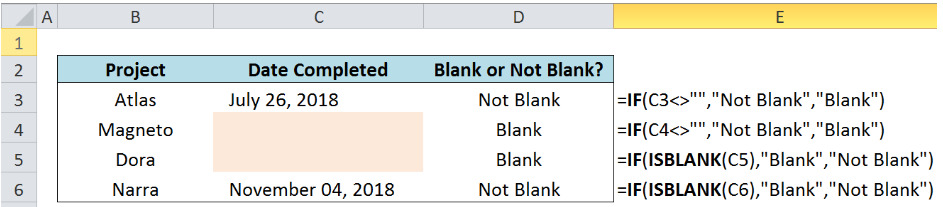Figure 1.  Final result: Determine if a cell is not blank in Excel

## IF Function in Excel

IF function evaluates a given logical test and returns a TRUE or a FALSE

### Syntax

`=IF(logical_test, [value_if_true], [value_if_false])`

• The arguments “value_if_true” and “value_if_false” are optional.  If left blank, the function will return TRUE if the logical test is met, and FALSE if otherwise.

## ISBLANK Function in Excel

ISBLANK function is more straightforward.  It tests whether a value or a cell is blank or not.

### Syntax

`=ISBLANK(value)`

• The function returns TRUE if the value is blank; FALSE if otherwise

## Setting up the Data

Below is a list of Projects and a column for Date Completed.  We want to know if column C for the “Date Completed” is blank or not.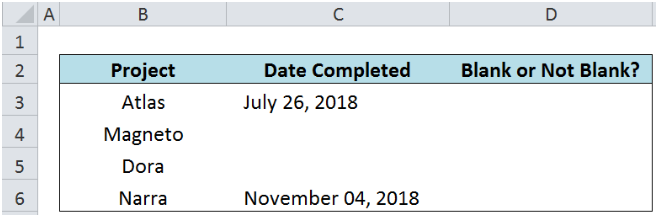Figure 2.  Sample data to determine if a cell is not blank

## Determine If a Cell is Not Blank

### Using the IF function

In cell D3, enter the formula:

`=IF(C3<>"","Not blank","Blank")`

• The symbol <> in Excel means “not equal to
• “” in Excel means empty string, or blank
• C3<>”” means C3 is not equal to blank, or C3 is not blank

This formula returns “Not blank” because cell C3 is not blank.  It has the value “July 26, 2018”.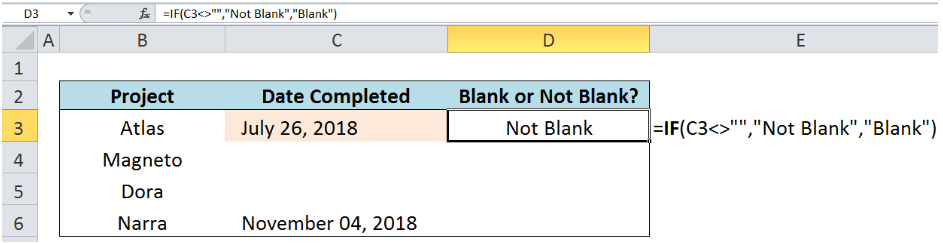Figure 3.  Entering the IF formula to determine if a cell is not blank

In cell D4, enter the formula:

`=IF(C4<>"","Not blank","Blank")`

The result is “Blank” because cell D4 is empty.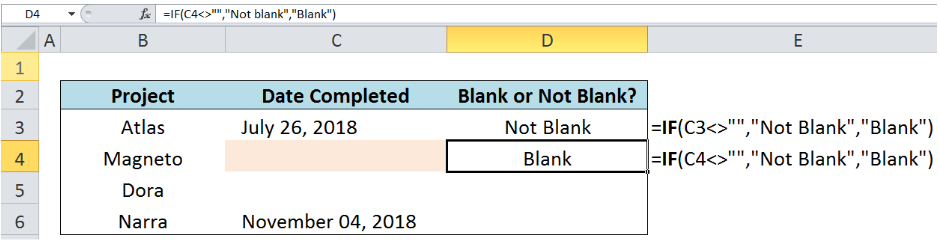Figure 4.  Output: Using IF to determine if a cell is not blank

### Using the IF and ISBLANK function

In cell D5, enter the formula:

`=IF(ISBLANK(C5),"Blank","Not Blank")`

• ISBLANK evaluates whether cell C5 is blank or not
• If the ISBLANK function returns TRUE, the formula will return “Blank”; otherwise, it returns “Not Blank”
• The result is “Blank” because C5 is empty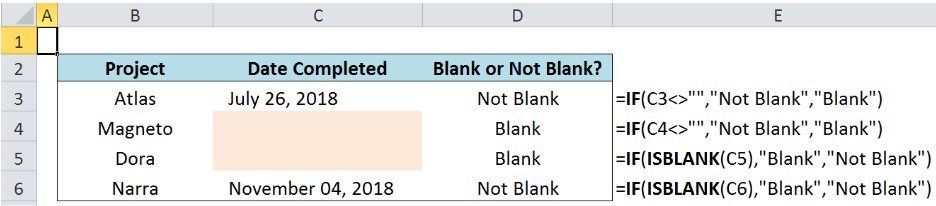Figure 5.  Final result: Using IF and ISBLANK to determine if a cell is not blank

## Note

We can customize the action that the IF function will return depending on the logical test results.

## Example

We want to return the status “Completed” if the cell in column C is not blank, and “In Progress” if otherwise.

Enter the following formula:

In cell D3:  `=IF(C3<>"","Completed","In Progress")`

In cell D4:  `=IF(C4<>"","Completed","In Progress")`

In cell D5:  `=IF(ISBLANK(C5),"In Progress","Completed")`

In cell D6:  `=IF(ISBLANK(C6),"In Progress","Completed")`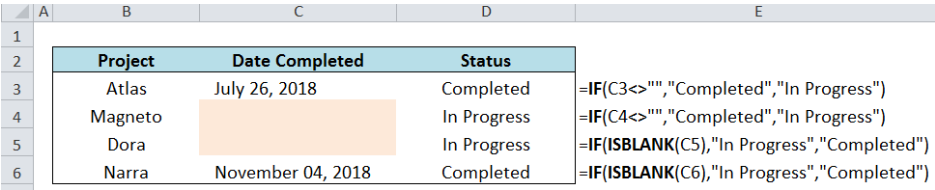Figure 6.  Final result: Using IF and ISBLANK to determine the status of a project

Most of the time, the problem you will need to solve will be more complex than a simple application of a formula or function. If you want to save hours of research and frustration, try our live Excelchat service! Our Excel Experts are available 24/7 to answer any Excel question you may have. We guarantee a connection within 30 seconds and a customized solution within 20 minutes.

Are you still looking for help with the IF function? View our comprehensive round-up of IF function tutorials here.

### Did this post not answer your question? Get a solution from connecting with the expert.Another blog reader asked this question today on Excelchat:## Subscribe to Excelchat.coAnother blog reader asked this question today on Excelchat: